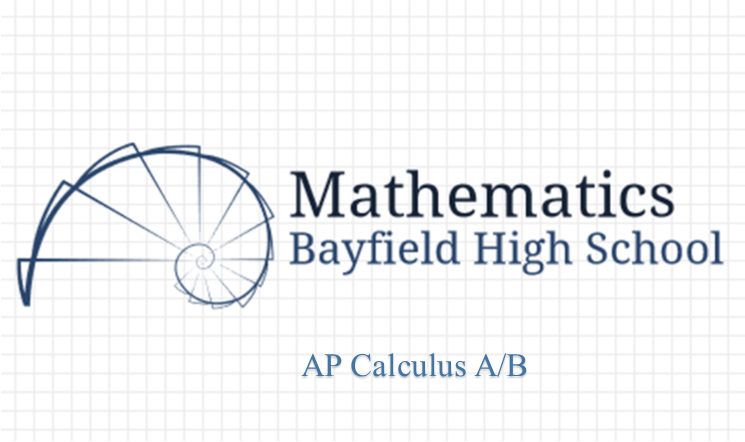# AP Calculus A/BCourse Description

AP Calculus A/B is primarily concerned with developing the students’ understanding of the concepts of calculus and with providing experience with its methods and applications. The course emphasizes a multi-representational approach to calculus, with concepts, results, and problems expressed graphically, numerically, analytically, and verbally. Understanding the connections among these representations is of primary importance.

As a result of this course, students should understand the meaning of the derivative in terms of a rate of change and as a local linear approximation. Students should understand the meaning of the definite integral both as a limit of Riemann sums and as the net accumulation of change. Students should understand the relationship between the derivative and the definite integral as expressed in both parts of the Fundamental Theorem of Calculus. Students should be able to solve a variety of problems that rely on the derivative and on the integral and should be able to distinguish between the two types of problems. It is the goal of the course to provide for the students sufficient knowledge of the subject so they can take the AP calculus test in the spring and score at least a 3 on the exam. A graphing calculator (such as the TI-83/84) is required. This class is reserved for juniors and seniors.

Prerequisite: Trig/Functions

Class Announcements

Supplies Needed Students will need a graph paper composition notebook, loose-leaf paper and 3 ring binder, pencil, and graphing calculator for each class day. Composition notebooks can be purchased from the math department for \$2 and calculators can be rented from the department on a first come first serve basis (\$20/year or \$0.50/class period for graphing calculator). We highly encourage students to buy their own because we have a limited number.